Home > Misc

# Tweet graphs

Because the unexamined life isn't worth tweeting.

Twitter allows users to download an archive of their tweets (from near the bottom of the Settings page; it might take a few minutes before a link is emailed to you). The zip file that Twitter creates for you contains both an HTML file with tweets indexed by month, and a CSV file with all of your tweets and retweets in one big table. We can load the latter into R and extract some statistics from it. (My code is at the end of this page; my regex is pretty rudimentary, but it seems to work for me.)

I thought it would be interesting to compare the frequencies of the words I tweet most with the frequencies from Google Ngrams (in what follows, I average the 1-gram frequencies over the period 1991-2000). I'll plug the ngramr package, which I wanted to use here, but because of a bug on Google's side I ended up writing my own function, borrowing fairly heavily from the ngramr source.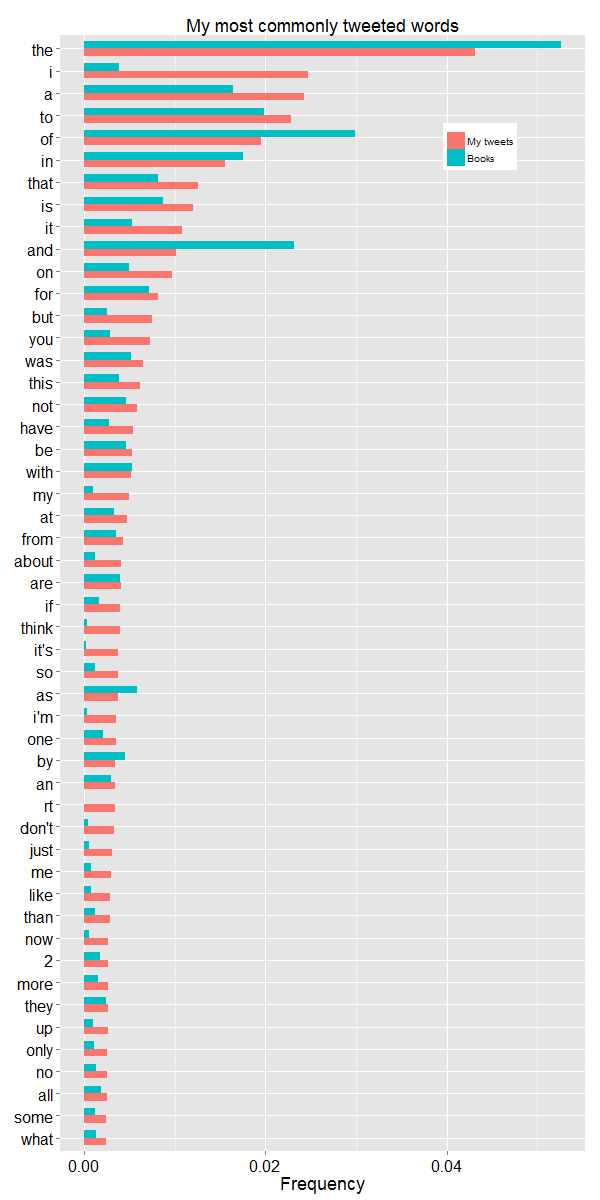The total word count for my tweets archive is 134167, so 1-standard-error error bars for a word with observed frequency 0.04 would be plus or minus sqrt[(0.04)(0.96)/134167] ≈ 0.0005.

Some of the differences between my tweets and Google's corpus are not surprising – I am more fond of personal pronouns than the average book, and I also use contractions more often. More curious is the difference between definite and indefinite articles. The latter are somewhat less common in my tweets than in the books, and the former are somewhat more common in my tweets than in the books. I don't have any intuition for why 'of' is so relatively rare in my tweets; the relative rarity of 'and' is perhaps due to Twitter's character limit, but I haven't investigated whether or not I often just replace 'and' with a comma when tweeting (I occasionally use ampersands, but not enough to explain that discrepancy, I think).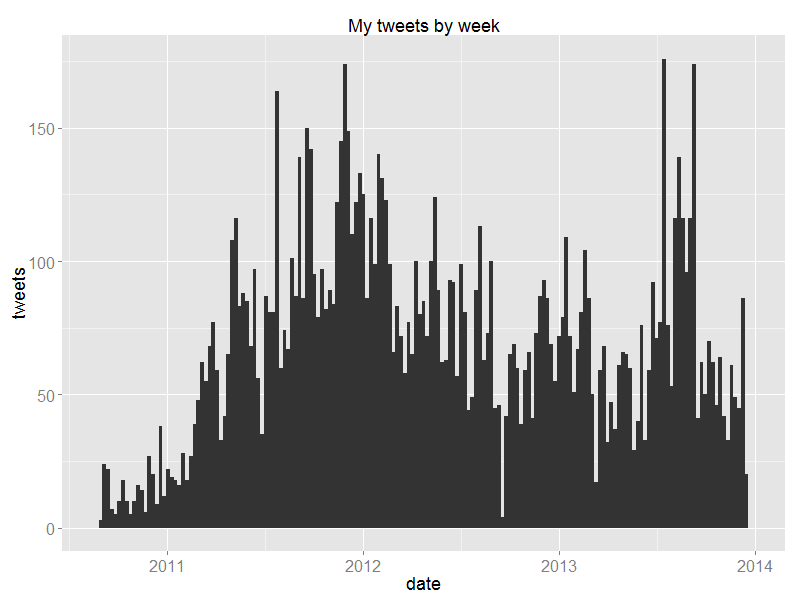It took me a few months to get into Twitter.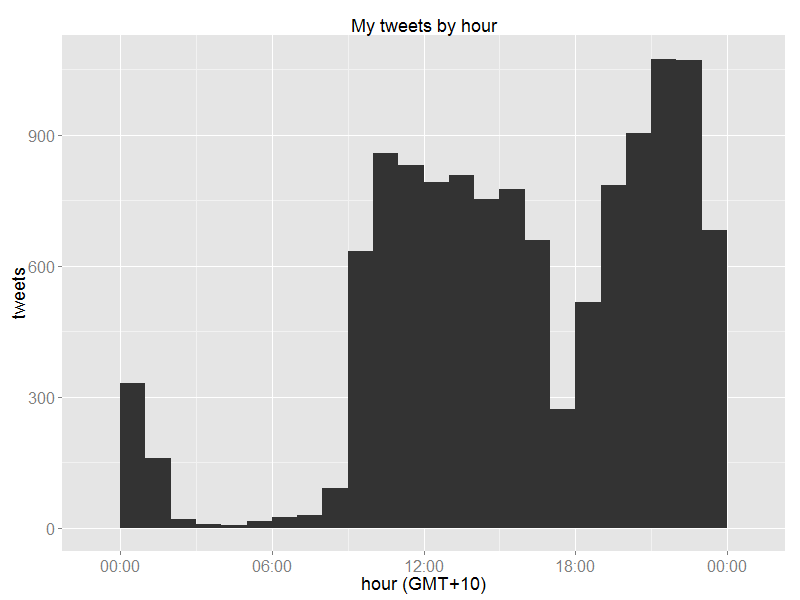My daily routine – the train ride home from work followed by cooking dinner make for a noticeable dip in Twitter activity.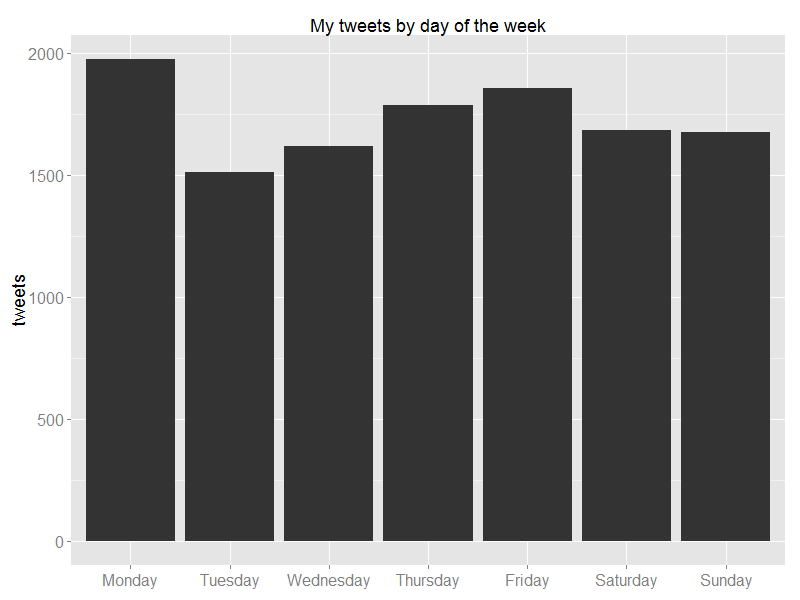More of my life reflected in tweet frequency: for most of my Twitter history I went to trivia nights on Tuesday evenings, and earlier this year I switched to Wednesdays.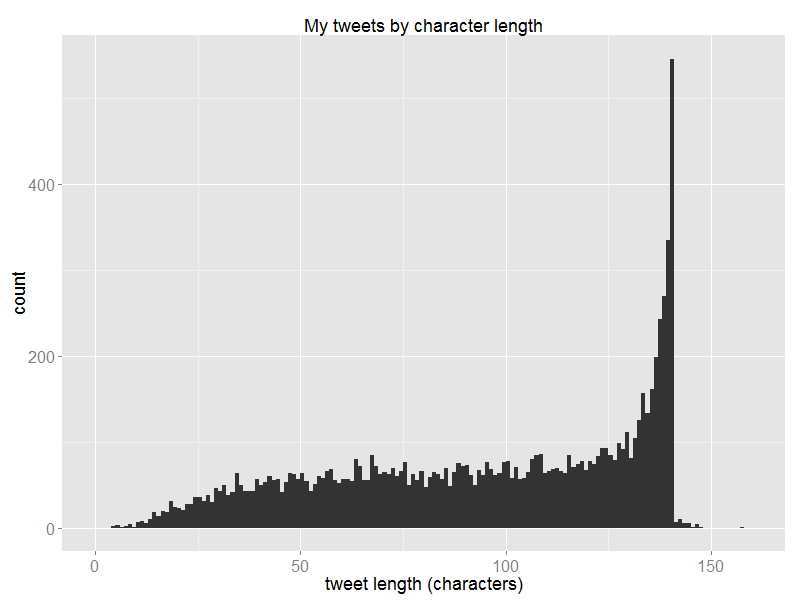I have a fairly strong belief that Twitter is successful as a medium for discussion and public debate because it enforces concision on the part of the authors, making life efficient for the readers (who are usually more numerous). I'm not alone running into the character limit often. (The outliers are caused by laziness on my part – the tweets.csv file stores the "<" character as "&lt;", and so forth, and I didn't convert these strings into the single-characters that they represent.)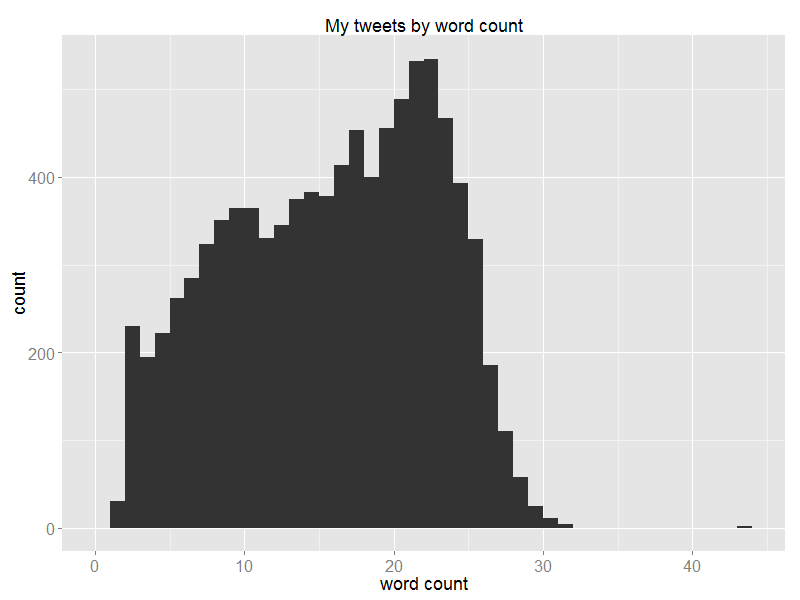A histogram by word count. The outlier on the far-right was me tweeting two-letter words at someone playing a game of Scrabble.

R code follows. Some of it looks a bit rough; it'd be a bit tidier were it not for the Google Ngrams apostrophe bug. I've only tested my code on my own tweets, which are almost exclusively in English; the regex will probably remove characters not in the English alphabet. You'll need to have wget installed. The whole thing only takes a few seconds to run, the longest part being the downloading of all the data from Google Ngrams.

``````library(ggplot2)
library(scales)
library(rjson)

# Hours ahead of GMT to convert to:
time_offset <- 10

# A function to print a plot to png:
png_print <- function(out_file, img_width, img_height, ggplot_img) {
png(filename=out_file, width=img_width, height=img_height, units="px")
print(ggplot_img)
dev.off()
return(T)
}

# At the time of writing, Google's Ngrams have a bug when a search term
# contains an apostrophe, which breaks the ngramr package, which relies
# on RCurl.  For reasons I don't understand, wget can follow a redirect
# to the correct URL containing the data, while cURL fails.
# So, rather than using ngramr, I wrote the following function to
# return the average ngram frequency over the given range (by default
# 1991-2000).  It requires wget to be installed.
ngram_average <- function(words, year_start=1991, year_end=2000, corpus=15) {
num_words <- length(words)
freqs <- rep(0, num_words)

words_replaced <- words

smoothing <- year_end - year_start + 1
words_plus <- gsub(" ", "+", words)

# Can only do 12 words at a time:
num_ngram_calls <- floor(num_words/12) + 1

for (ct in 1:num_ngram_calls) {
i1 <- (ct-1)*12 + 1
i2 <- min(ct*12, num_words)
words_subset <- words_plus[i1:i2]

words_str <- paste(words_subset, collapse=",")
words_str, year_start, year_end, smoothing, corpus)

wget_str <- sprintf("wget --no-check-certificate -O temp_wget.html %s", url)

system(wget_str)
file.remove("temp_wget.html")

replacement_lines <- html[grep("to match how we processed the books", html)]
num_replacements <- length(replacement_lines)
if (num_replacements > 0) {
replaced <- gsub(".*Replaced ", "", replacement_lines)
replaced <- gsub(".*", "", replaced)
replaced <- gsub("'", "'", replaced)

replacements <- gsub(".*with ", "", replacement_lines)
replacements <- gsub(".*", "", replacements)
replacements <- gsub("'", "'", replacements)

for (i in 1:num_replacements) {
word_index <- which(words == replaced[i])
if (length(word_index) == 0) {
# Hopefully not needed
print(sprintf("Couldn't find %s", replaced))
}
words_replaced[word_index] <- replacements[i]
}
}

data_line <- grep("var data", html)
json_str <- sub(".*= ", "", html[data_line])
ngram.list <- fromJSON(json_str)

# If one of the words searched for didn't return any ngrams, then
# it won't appear in the ngram data, so we have to match
# up the words in the ngram data to the correct entry in the
# vector of frequencies to be returned.
these_words <- sapply(ngram.list, function(x) x\$ngram)
these_freqs <- sapply(ngram.list, function(x) x\$timeseries)

these_words <- gsub("'", "'", these_words)

num_these_words <- length(these_words)
if (num_these_words > 0) {
for (i in 1:num_these_words) {
word_index <- which(words_replaced == these_words[i])
if (length(word_index) == 0) {
# Hopefully not needed
print(sprintf("Couldn't find the word %s", these_words[i]))
}
freqs[word_index] <- these_freqs[i]
}
}
}

return(freqs)
}

tweet.df\$datetimes <- as.POSIXct(sub(" [^ ]*\$", "", tweet.df\$timestamp))
tweet.df\$datetimes <- tweet.df\$datetimes + 3600*time_offset
# Strip the date for getting time of day:
tweet.df\$times <- as.POSIXct(strftime(tweet.df\$datetimes,
format="%H:%M:%S"),
format="%H:%M:%S")

tweet.df\$days <- factor(weekdays(tweet.df\$datetimes),
levels=c("Monday", "Tuesday", "Wednesday", "Thursday",
"Friday", "Saturday", "Sunday"),
ordered=T)

# When doing analyses of words/characters, remove retweets
# (not perfect, since it'll retain manual RT's, but the
# non-manual RT's often have length 142...):
nonRT <- which(is.na(tweet.df\$retweeted_status_id))
nonRT_tweet.df <- tweet.df[nonRT, ]

nonRT_tweet.df\$chars <- nchar(nonRT_tweet.df\$text)

# When counting word frequencies, we want to remove links, so that
# strings like "http", "co", etc. don't appear amongst the most
# tweeted words.  The column text2 will be added to nonRT_tweet.df
# for this purpose.
#
# When doing a word-count in a tweet, we want to treat the link
# as one word.  The column text3 will be added to nonRT_tweet.df
# for this purpose.

# Replace links with spaces; replace most non-word characters with spaces;
# condense multiple spaces to single space:
nonRT_tweet.df\$text2 <- nonRT_tweet.df\$text
nonRT_tweet.df\$text2 <- gsub("https?:[^ ]*", " ", nonRT_tweet.df\$text2)
nonRT_tweet.df\$text2 <- gsub("[^ ]*\\.(co|org|net|ly)[^ ]*", " ", nonRT_tweet.df\$text2)
nonRT_tweet.df\$text2 <- gsub("[^A-Za-z0-9\'@#_]", " ", nonRT_tweet.df\$text2)
nonRT_tweet.df\$text2 <- gsub(" +", " ", nonRT_tweet.df\$text2)

# As above, but replace links with the word "link" and trim leading/trailing spaces:
nonRT_tweet.df\$text3 <- nonRT_tweet.df\$text
nonRT_tweet.df\$text3 <- gsub("https?:[^ ]*", "link", nonRT_tweet.df\$text3)
nonRT_tweet.df\$text3 <- gsub("[^ ]*\\.(co|org|net|ly)[^ ]*", "link", nonRT_tweet.df\$text3)
nonRT_tweet.df\$text3 <- gsub("[^A-Za-z0-9\'@#_]", " ", nonRT_tweet.df\$text3)
nonRT_tweet.df\$text3 <- gsub(" +", " ", nonRT_tweet.df\$text3)
nonRT_tweet.df\$text3 <- gsub(" +\$", "", nonRT_tweet.df\$text3)
nonRT_tweet.df\$text3 <- gsub("^ +", "", nonRT_tweet.df\$text3)

# tweet_words is used to count word frequencies (1-grams).
tweet_words <- tolower(unlist(strsplit(nonRT_tweet.df\$text2, " ")))
tweet_words <- tweet_words[-which(tweet_words=="")]
tweet_words <- tweet_words[-grep("@", tweet_words)]
tweet_words <- factor(tweet_words)

# Idea to count words is to count spaces and add 1.  But the gregexpr will return
# -1 if there are no spaces, which has length 1, and so a naive "length + 1"
# algorithm will say that the string "blah" contains two words.
nonRT_tweet.df\$firstspace <- sapply(gregexpr(" ", nonRT_tweet.df\$text3), function(x) x)
single_words <- which(nonRT_tweet.df\$firstspace == -1)

nonRT_tweet.df\$wordcount <- sapply(gregexpr(" ", nonRT_tweet.df\$text3), length) + 1
# Now over-ride those values when it's a single word:
nonRT_tweet.df\$wordcount[single_words] <- 1

num_summary_words <- 50
word_freqs <- summary(tweet_words, maxsum=num_summary_words+1)
word_freqs_nonother <- word_freqs[1:num_summary_words]
freq.df <- data.frame(words=factor(names(word_freqs_nonother),
levels=names(word_freqs_nonother)[num_summary_words:1],
ordered=T),
count=word_freqs_nonother,
freq=word_freqs_nonother/sum(word_freqs),
word_source="My tweets")

words_char <- as.character(freq.df\$words)
ngram_freqs <- ngram_average(words_char)
# Re-do the ngrams with a capital letter:
words_char <- sub("([a-z])", "\\U\\1", words_char, perl=T)
ngram_freqs <- ngram_freqs + ngram_average(words_char)

ngram_freq.df <- freq.df
ngram_freq.df\$word_source <- "Books"
ngram_freq.df\$freq <- ngram_freqs

freq.df <- rbind(freq.df, ngram_freq.df)

base_plot <- ggplot() + theme(axis.title.x = element_text(size=18),
axis.text.x = element_text(size=16),
axis.title.y = element_text(size=18),
axis.text.y = element_text(size=16),
plot.title=element_text(size=18))

date_plot <- base_plot + geom_histogram(data=tweet.df,
aes(x=datetimes),
binwidth=7*86400) +
xlab("date") + ylab("tweets") + ggtitle("My tweets by week")

time_plot <- base_plot + geom_histogram(data=tweet.df,
aes(x=times),
binwidth=3600) +
xlab(sprintf("hour (GMT+%d)", time_offset)) + ylab("tweets") +
ggtitle("My tweets by hour") +
scale_x_datetime(labels = date_format("%H:%M"))

day_plot <- base_plot + geom_bar(data=tweet.df, aes(x=days)) +
ylab("tweets") + ggtitle("My tweets by day of the week") +
theme(axis.title.x = element_blank())

wordfreq_plot <- base_plot + geom_bar(data=freq.df,
aes(x=words,
y=freq,
fill=word_source,
width=0.67),
stat="identity",
position="dodge") +
ylab("Frequency") + xlab("") + ggtitle("My most commonly tweeted words") +
theme(axis.text = element_text(colour="#000000"),
axis.title.y = element_blank(),
legend.title = element_blank(),
legend.position = c(0.8, 0.9)) +
coord_flip()

chars_plot <- base_plot + geom_histogram(data=nonRT_tweet.df,
aes(x=chars),
binwidth=1) +
xlim(0, 160) + xlab("tweet length (characters)") +
ggtitle("My tweets by character length")

wordcount_plot <- base_plot + geom_histogram(data=nonRT_tweet.df,
aes(x=wordcount),
binwidth=1) +
xlab("word count") + ggtitle("My tweets by word count")

png_print("histogram_date.png", 800, 600, date_plot)
png_print("histogram_time.png", 800, 600, time_plot)
png_print("histogram_charlength.png", 800, 600, chars_plot)
png_print("histogram_wordcount.png", 800, 600, wordcount_plot)
png_print("word_freqs.png", 600, 1200, wordfreq_plot)
png_print("dayofweek.png", 800, 600, day_plot)```
```

Home > Misc